I know I'm not supposed to ask 2 questions but if you could please help me on these questions it would help me so much and i will thank you endlessly, i have 2 questions left that i am stuck on but only one question left for chegg, I thank you so much in advance and I wish nothing but health and happiness for all you.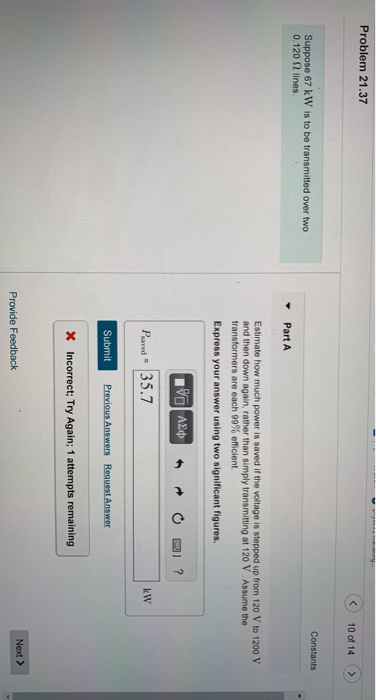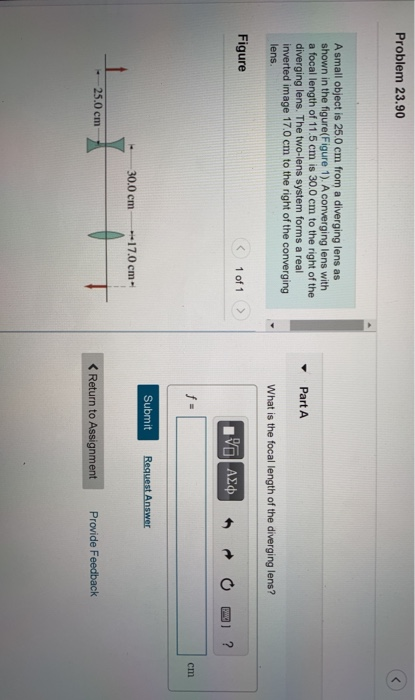Problem 21.37 10 of 14 Constants Suppose 67 kW is to be transmitted over two 0.120 2 lines y Part A Estimate how much power is saved if the voltage is stepped up from 120 V to 1200 V and then down again, rather than simply transmitting at 120 V Assume the transformers are each 99% efficient Express your answer using two significant figures. Pal AΣΦ. ? Psaved - 35.7 kW Submit Previous Answers Request Answer X Incorrect; Try Again; 1 attempts remaining Next > Provide Feedback
Problem 23.90 A small object is 25.0 cm from a diverging lens as shown in the figure(Figure 1). A converging lens with a focal length of 11.5 cm is 30.0 cm to the right of the diverging lens. The two-lens system forms a real inverted image 17.0 cm to the right of the converging lens Part A What is the focal length of the diverging lens? Figure 1 of 1 ΟΙ ΑΣΦ ? f- cm Submit Request Answer 30.0 cm 17.0 cm 25.0 cm t < Return to Assignment Provide Feedback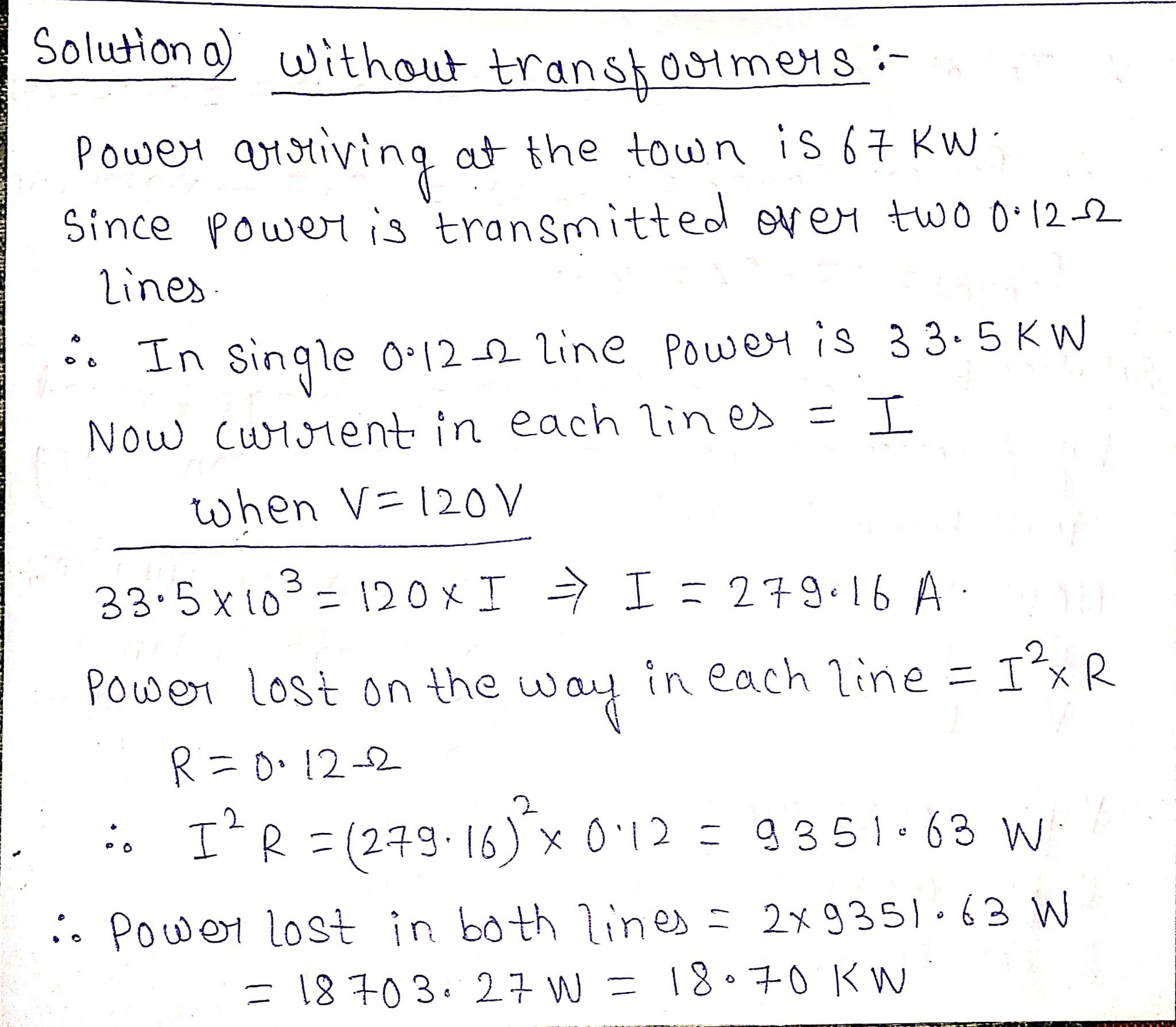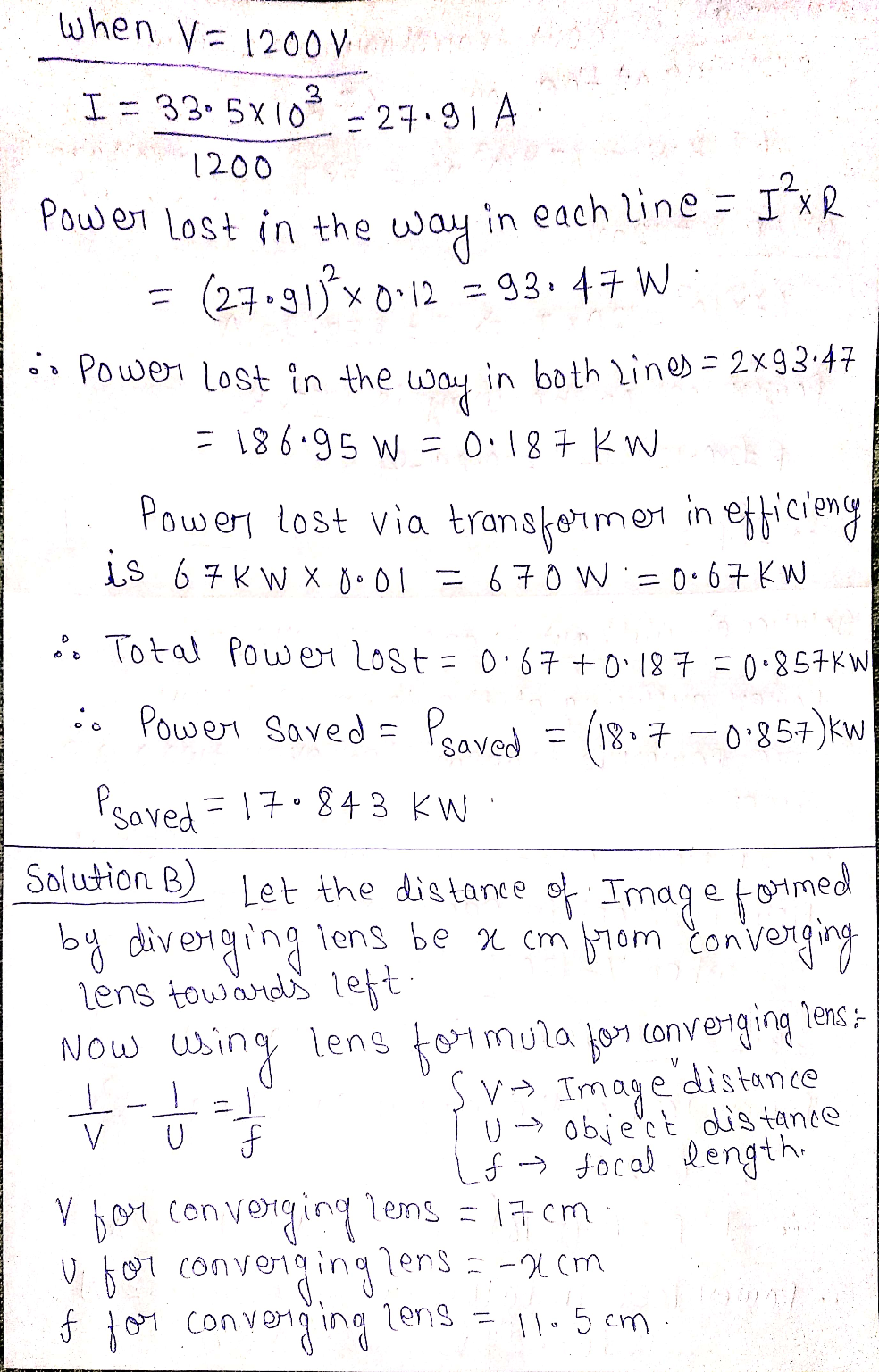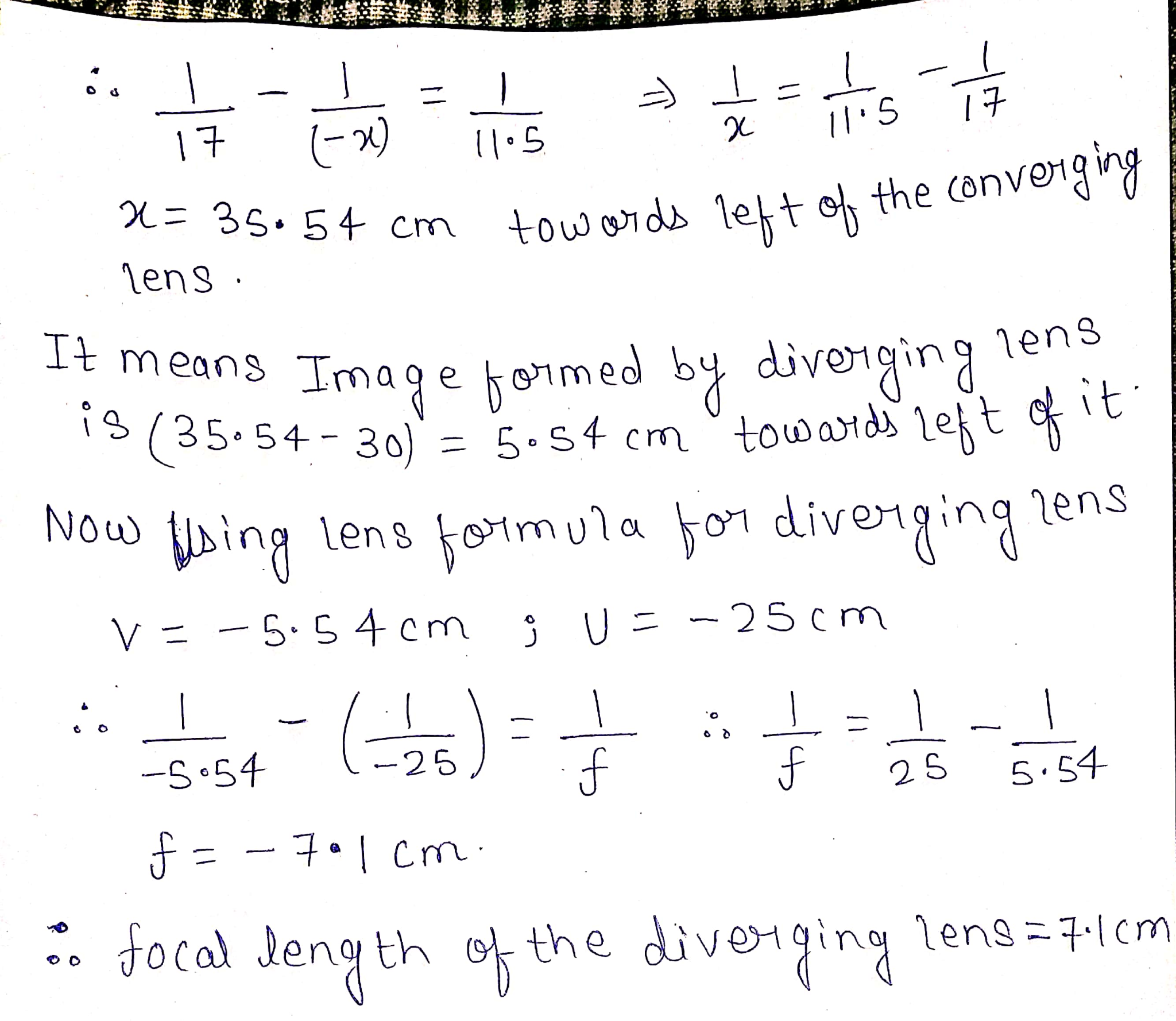#### Earn Coin

Coins can be redeemed for fabulous gifts.

Similar Homework Help Questions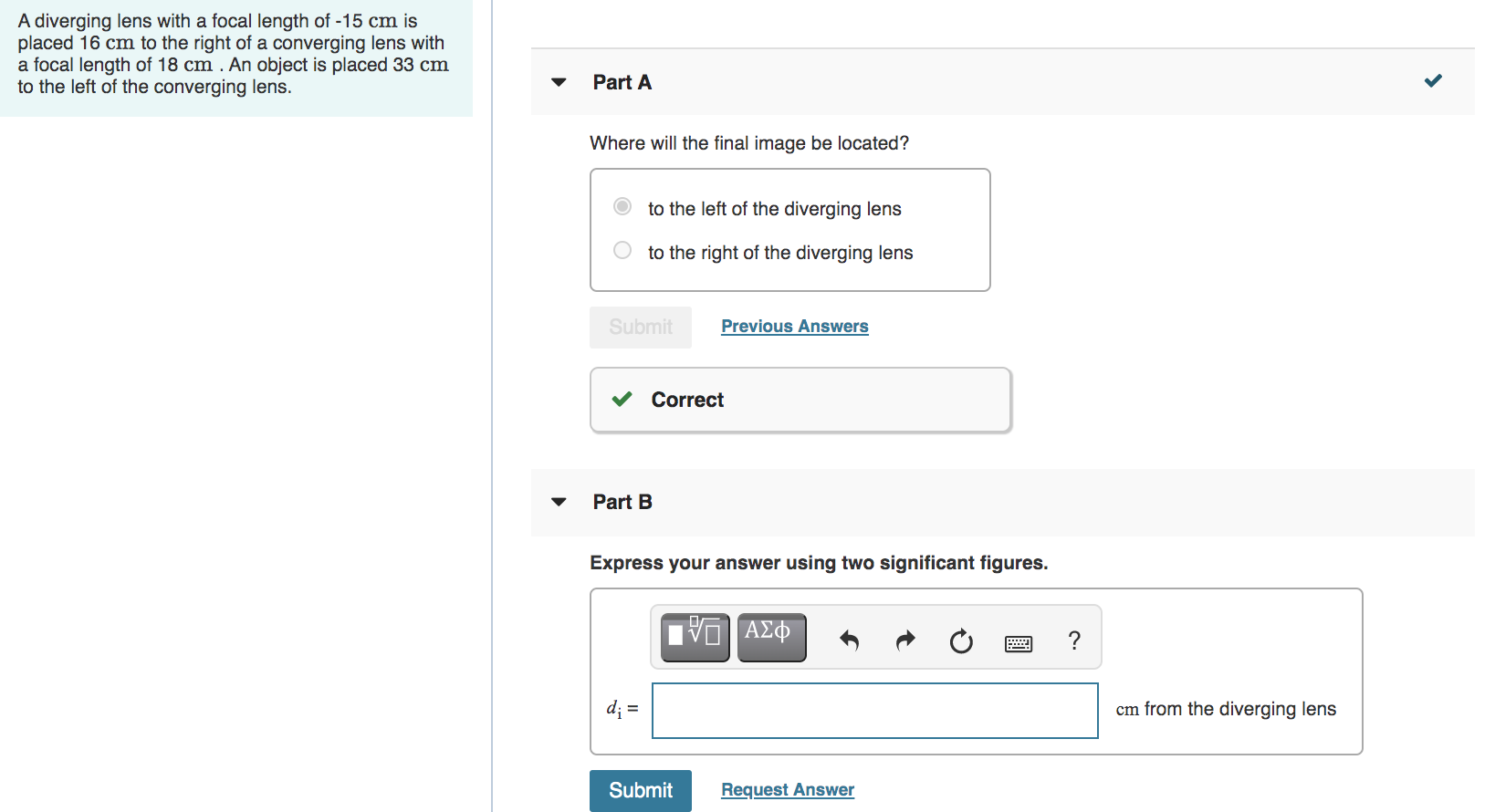Please HELP!!! I will rate good for good answers! A diverging lens with a focal length of -15 cm is placed 16 cm to the right of a converging lens with a focal length of 18 cm . An object is placed 33 cm to the left of the converging lens. Part A Where will the final image be located? to the left of the diverging lens to the right of the diverging lens Submit Previous Answers Correct Part B...

• ### I have no idea how to do this. Will you please work it out and write...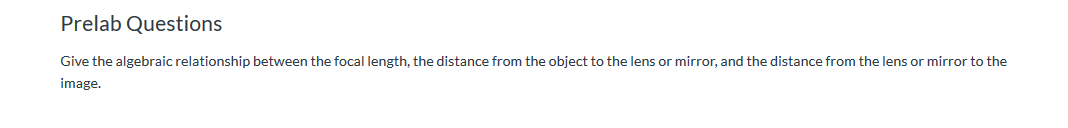I have no idea how to do this. Will you please work it out and write neat enough to read? Thank you! Prelab Questions Give the algebraic relationship between the focal length, the distance from the object to the lens or mirror, and the distance from the lens or mirror to the image. Thick Converging Lens As in part 1 of this lab, we're going to measure the focal length of a converging lens - a lens that is thicker...

• ### please help me with two questions thank youu 1. An object of 5 cm height is...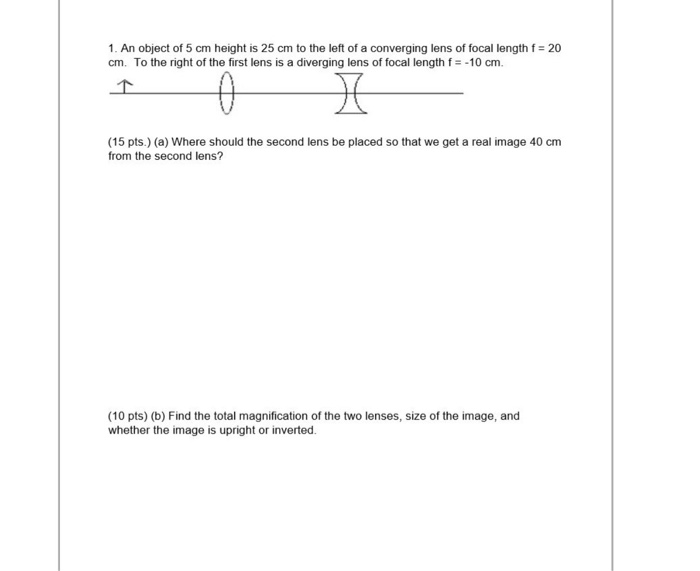please help me with two questions thank youu 1. An object of 5 cm height is 25 cm to the left of a converging lens of focal length f = 20 cm. To the right of the first lens is a diverging lens of focal length f = -10 cm. P M (15 pts.) (a) Where should the second lens be placed so that we get a real image 40 cm from the second lens? (10 pts) (b) Find the...

• ### Please provide specific explanations for each question. I do not know any materials for these questions,...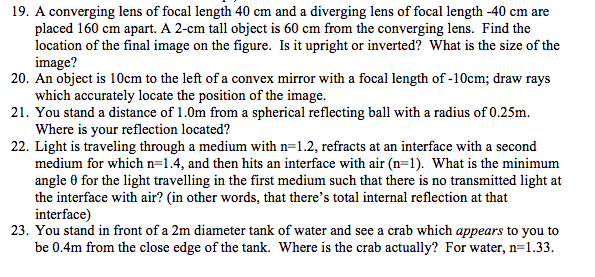Please provide specific explanations for each question. I do not know any materials for these questions, so please teach me specifically and professionally. Thank you a lot. Also, clear handwriting notes are important! 19. A converging lens of focal length 40 cm and a diverging lens of focal length -40 cm are placed 160 cm apart. A 2-cm tall object is 60 cm from the converging lens. Find the location of the final image on the figure. Is it upright...

Physics Ray Optics Lab: (please help and answer asap/ask if there are additional questions) I just need help with part F for the bolded questions. Part E: In step 6 of Part E of the experimental procedure, you measured a combined or effective focal length of a converging and diverging glass lens. When two lenses are used together, they have an effective focal length eff Data: Part E Clear Converging Lens 12 cm Diverging + Clear Converging Lens 15 cm...

• ### Could you please show all of the steps taken in solving the problem and explain the process fully...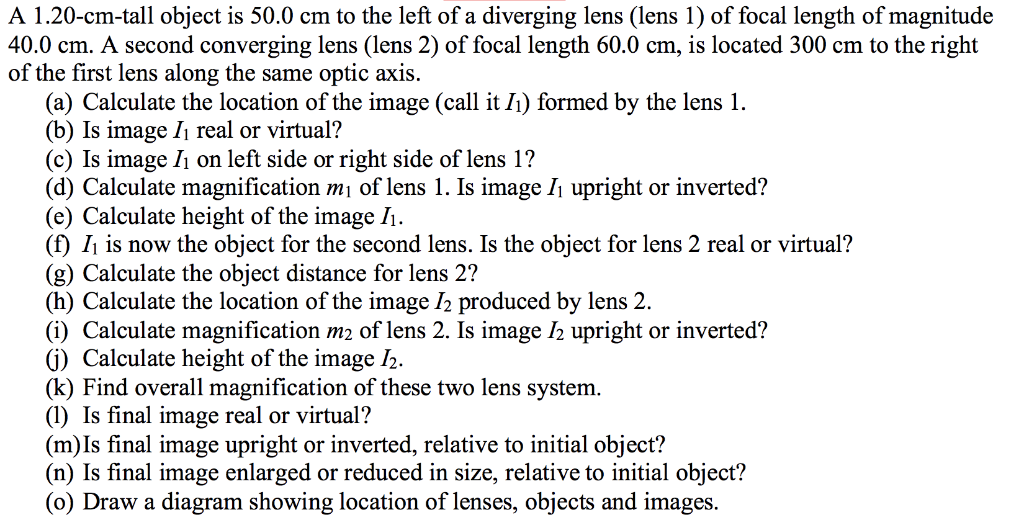Could you please show all of the steps taken in solving the problem and explain the process fully, using sentences/phrases (if possible) to understand why those steps were taken and why you got that answer. Also could you please draw a diagram with any applicable variables/values to help visualize the problem better. Thank you in advance for all the help! A 1.20-cm-tall object is 50.0 cm to the left of a diverging lens (lens 1) of focal length of magnitude...

• ### please I need answers to all questions because am writing text on Friday. 7. Suppose an...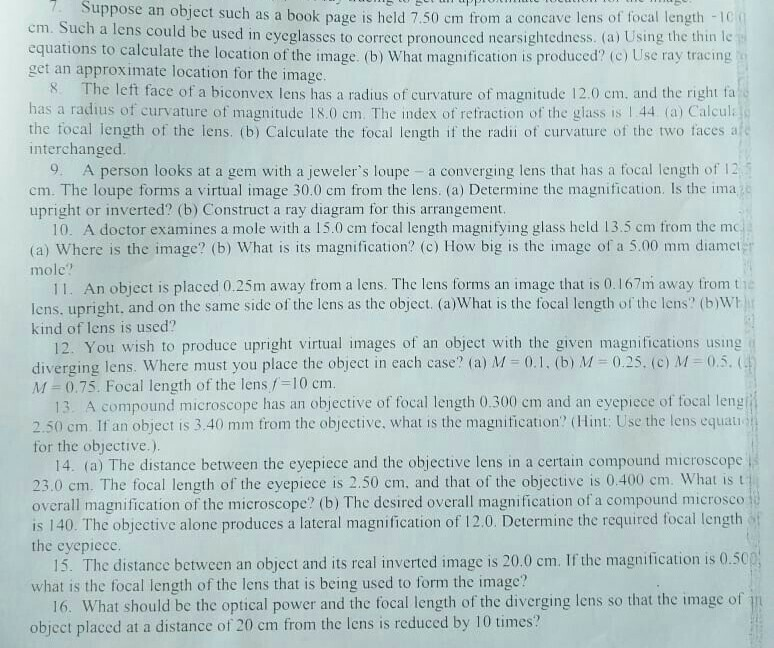please I need answers to all questions because am writing text on Friday. 7. Suppose an object such as a book page is held 7.50 cm from a concave lens of focal length -1C em. Such a lens could be used in eyeglasses to correct pronounced nearsightedness. (a) Using the thin le equations to calculate the location of the image. (b) What magnification is produced? (c) Use ray tracing get an approximate location for the image. 8. The left face...

• ### Pleas help me with both parts I don't know how to do this. Thank u Constants...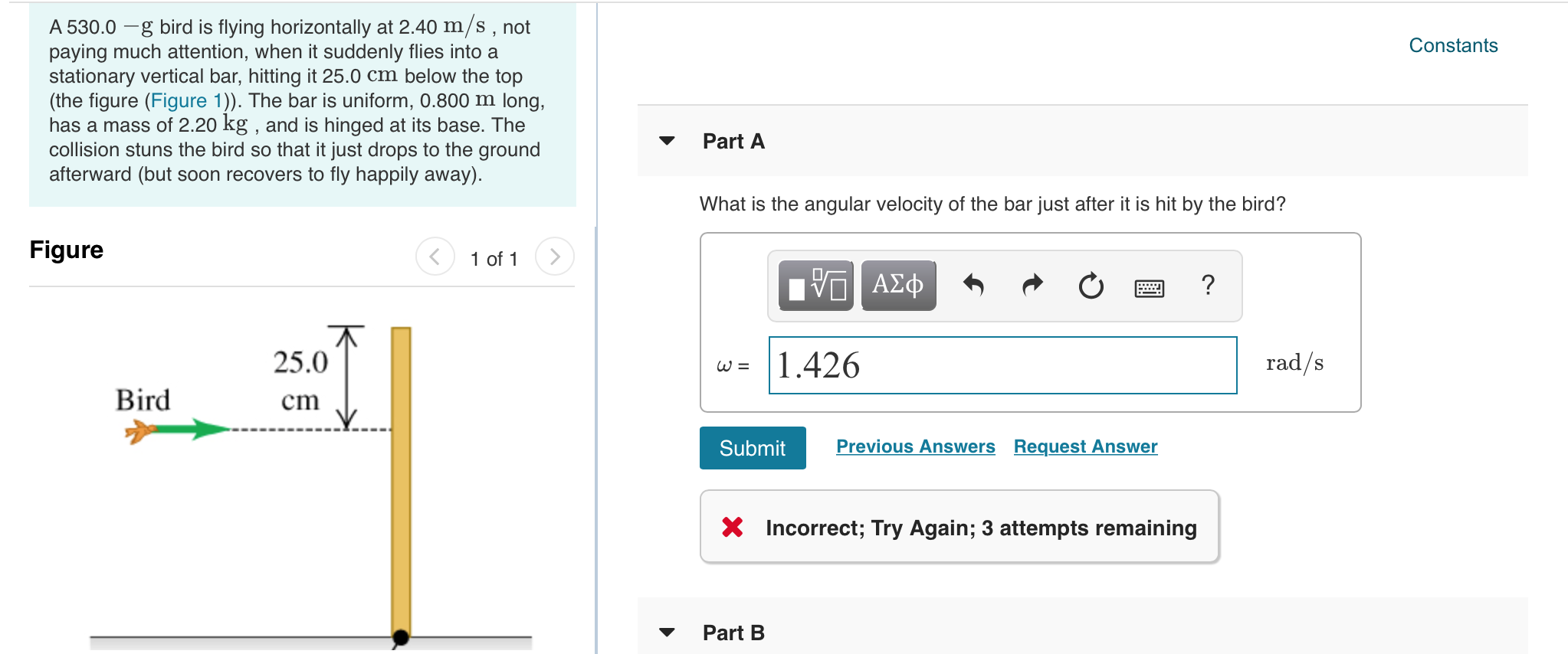Pleas help me with both parts I don't know how to do this. Thank u Constants A 530.0 -g bird is flying horizontally at 2.40 m/s, not paying much attention, when it suddenly flies into a stationary vertical bar, hitting it 25.0 cm below the top (the figure (Figure 1)). The bar is uniform, 0.800 m long, has a mass of 2.20 kg , and is hinged at its base. The collision stuns the bird so that it just drops...

• ### Please answer questions 1 & 2. I got 214.29 cm for Q1 and for Q2 I...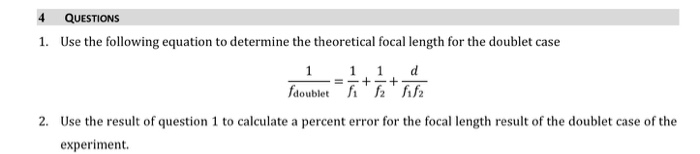Please answer questions 1 & 2. I got 214.29 cm for Q1 and for Q2 I got 92.41% in error. Can you confirm if these answers are correct? Thank you! This is my calculated data from using the formula 2 for the focal length of doublet 4 QUESTIONS 1. Use the following equation to determine the theoretical focal length for the doublet case 1 1 1 d = - + - + fdoublett oublet 2. Use the result of question...

• ### Need help with part B. Thank you! <2303 Fall 2019 Homework 4 Exercise 34.41 - Enhanced...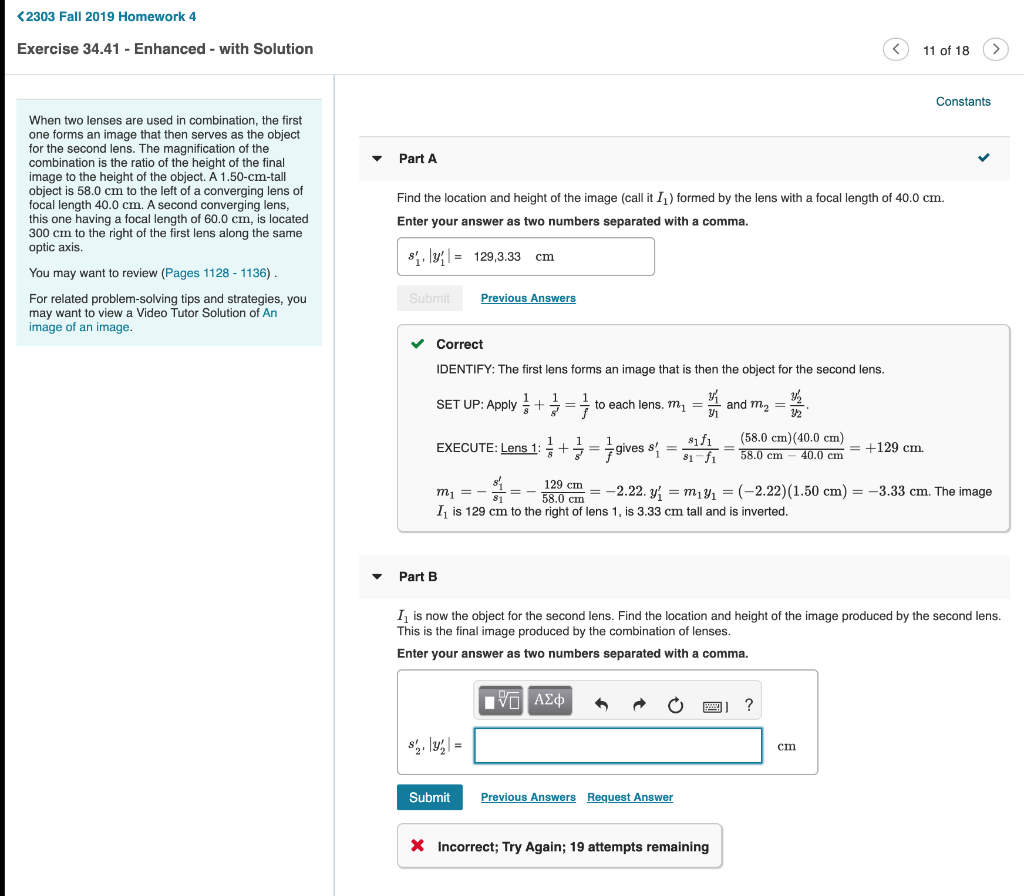Need help with part B. Thank you! <2303 Fall 2019 Homework 4 Exercise 34.41 - Enhanced - with Solution 11 of 18 > Constants Part A When two lenses are used in combination, the first one forms an image that then serves as the object for the second lens. The magnification of the combination is the ratio of the height of the final image to the height of the object. A 1.50-cm-tall object is 58.0 cm to the left of...